Next: N finite slits: combining Up: Finite slit: Diffraction Previous: Analysis

## Interpretation

Figure 9 shows an example of a diffraction pattern from a single slit. Again, several features are noteworthy.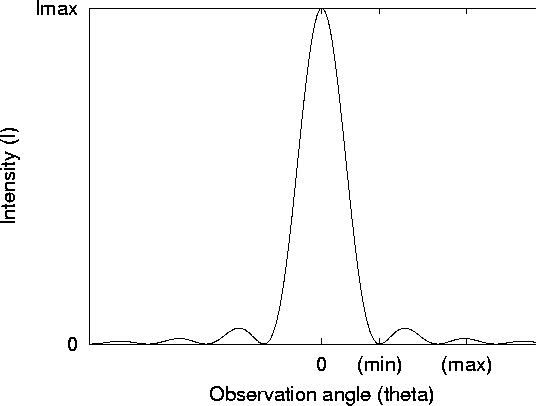Figure 9: Intensity as a function of observation angle for a finite-slit experiment

• A single, central ``principle maximum'': From the discussion for the N-slit pattern, we only see principle maxima after every N-1 minima, where N is the number of slits. Because we now have an effectively infinite number of slits, we only see the central principle maximum at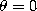.
• Minima: Minima occur whenever the numerator is zero (and the denominator is not). This happens whenever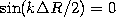, equivalently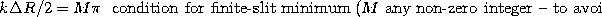This corresponds to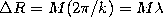, a path difference between one end of the slit and the other of any non-zero integer number of wavelengths. If the path difference is zero, all the contributions interference constructively, and you get a large maximum. If the path difference is not zero, but an even number of wavelengths, then we see that you always find exactly canceling negative and positive contributions from difference regions of the slit, leading ultimately to a minimum.

• Lesser Maxima: Again, there will be lesser maxima situated approximately halfway in between the minima.

Tomas Arias
Thu Sep 13 15:26:14 EDT 2001